Thank you for visiting nature.com. You are using a browser version with limited support for CSS. To obtain the best experience, we recommend you use a more up to date browser (or turn off compatibility mode in Internet Explorer). In the meantime, to ensure continued support, we are displaying the site without styles and JavaScript.

# Quantum principal component analysis

## Abstract

The usual way to reveal properties of an unknown quantum state, given many copies of a system in that state, is to perform measurements of different observables and to analyse the results statistically1,2. For non-sparse but low-rank quantum states, revealing eigenvectors and corresponding eigenvalues in classical form scales super-linearly with the system dimension3,4,5,6. Here we show that multiple copies of a quantum system with density matrix ρ can be used to construct the unitary transformation eiρt. As a result, one can perform quantum principal component analysis of an unknown low-rank density matrix, revealing in quantum form the eigenvectors corresponding to the large eigenvalues in time exponentially faster than any existing algorithm. We discuss applications to data analysis, process tomography and state discrimination.

## Main

Quantum tomography is the process of discovering features of an unknown quantum state ρ (refs 1, 2). Quantum tomography is a widely used tool with important practical applications in communication systems such as optical channels, precision measurement devices such as atomic clocks, and quantum computation. The basic assumption of quantum tomography is that one is given multiple copies of ρ in a d-dimensional Hilbert space, for example, states of atoms in an atomic clock or inputs and outputs of a quantum channel. A variety of measurement techniques allow one to extract desired features of the state. For example, recent developments have shown that quantum compressed sensing can give significant advantages for determining the unknown state or dynamics of a quantum system, particularly when that state or dynamics can be represented by low-rank or sparse matrices3,4,5,7,8. The spectral decomposition of a matrix represents the matrix in terms of its eigenvectors and corresponding eigenvalues. The optimal low-rank approximation of a matrix can be constructed from the spectral decomposition by discarding the eigenvalues and corresponding eigenvectors below a given threshold. This procedure is frequently called principal component analysis (PCA), and can be used, for example, to construct the low-rank approximation of the positive semidefinite symmetric covariance matrix of sampled random vectors9,10. The cost of PCA is prohibitive when one is presented with a large number of high-dimensional vectors6.

Conventional quantum state tomography operates by making measurements on multiple copies of the state: the state plays a passive role. This paper shows that the state can play a dynamic role in its own analysis. In particular, we show that multiple copies of the state ρ can be used to implement the unitary operator eiρt: that is, the state functions as an energy operator or Hamiltonian, generating transformations on other states. Thus, multiple copies of a density matrix can be used to reveal the matrix’s eigenvectors and eigenvalues in quantum form. With further processing and measurements, this density matrix exponentiation can provide significant advantages for quantum tomography. Moreover, it allows us to perform quantum PCA (qPCA) of an unknown low-rank density matrix to construct the eigenvectors corresponding to the large eigenvalues of the state (the principal components) in time O(log d), an exponential speed-up over existing algorithms. We also show how qPCA can provide new methods of state discrimination and cluster assignment.

Suppose that one is presented with n copies of ρ. A simple trick allows one to apply the unitary transformation eiρt to any density matrix σ up to nth order in t. Note that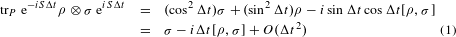Here trP is the partial trace over the first variable and S is the swap operator. S is a sparse matrix and its elements are efficiently computable, so eiSΔt can be performed efficiently11,12,13,14. Repeated application of (1) with n copies of ρ allows one to construct eiρnΔtσeiρnΔt. Comparison with the Suzuki–Trotter theory of quantum simulation11,12,13,14 shows that to simulate eiρt to accuracy ε requires n = O(t2ε−1|ρ − σ|2) ≤ O(t2ε−1) steps, where t = nΔt and | | is the sup norm. Thus, simply performing repeated infinitesimal swap operations on ρ σ allows us to construct the unitary operator eiρt. The quantum matrix inversion techniques of ref. 15 then allow us to use multiple copies of a density matrix ρ to implement eig(ρ) efficiently for any simply computable function g(x).

Note that density matrix exponentiation is most effective when some of the eigenvalues of ρ are large. If all of the eigenvalues are of size O(1/d) then we require time t = O(d) to resolve the eigenvalues15. In contrast, if the density matrix is dominated by a few large eigenvalues—that is, when the matrix is well represented by its principal components—then the method works well (the accuracy will be analysed below). In this case, there exists a subspace of dimension R d such that the projection of ρ onto this subspace is close to ρ: ρ − PρP1 ≤ ε, where P is the projector onto the subspace. When the matrix is of low rank, the projection is exact. Present techniques for matrix exponentiation are efficient when the matrix to be exponentiated is sparse13,14. The construction here shows that non-sparse but low-rank matrices can also be exponentiated efficiently as long as multiple copies of the corresponding density matrix are available.

Density matrix exponentiation now allows us to apply the quantum phase algorithm to find the eigenvectors and eigenvalues of an unknown density matrix. If we have n copies of ρ, we use the ability to apply eiρt to perform the quantum phase algorithm1. In particular, the quantum phase algorithm uses conditional applications of eiρt for varying times t to take any initial state |ψ〉|0〉 to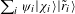, where |χi〉 are the eigenvectors of ρ,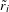are estimates of the corresponding eigenvalues, and ψi = 〈χi|ψ〉. In refs 16, 17 it was shown that the ability to apply an unknown unitary does not automatically translate into the ability to apply the unitary in a conditional fashion. Here, in contrast, the conditional operation can be performed simply by replacing the SWAP operator with a conditional SWAP in the derivation above. More precisely, taket = nΔt, and apply the unitary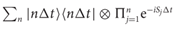to the state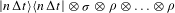where σ = |χ〉〈χ| and Sj swaps σ with the jth copy of ρ. Taking the partial trace over the copies of ρ yields the desired conditional operation |t〉|χ〉 → |t〉eiρt|χ〉. Inserting this conditional operation in the quantum phase algorithm and using the improved phase-estimation techniques of ref. 15 yields the eigenvectors and eigenvalues to accuracy ε by applying the quantum phase algorithm for time t = O(ε−1), and so requires n = O(1/ε3) copies of the state ρ. Using ρ itself as the initial state, the quantum phase algorithm yields the state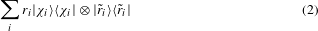Sampling from this state allows us to reveal features of the eigenvectors and eigenvalues of ρ. The use of multiple copies of a state to construct its eigenvectors and eigenvalues will be referred to here as qPCA.

As above, qPCA is useful if ρ has small rank R or admits a rank R approximation. In this case, only the largest R eigenvalues will register as non-zero in the eigenvector/eigenvalue decomposition (2). Using mn copies of ρ we obtain m copies of the decomposition (2), where the ith eigenvalue ri appears rim times. The features of the ith eigenstate can then be determined by performing a quantum measurement to obtain the expectation value 〈χi|M|χi〉 of the eigenvector with eigenvalue ri for desired Hermitian M. As long as M is sparse11,12,13,14 or efficiently simulable by the methods given in this paper, this measurement can itself be performed in time O(log d). qPCA efficiently reveals the eigenvectors and eigenvalues of the unknown density matrix ρ and allows one to probe their properties. For example, in many-body quantum systems in condensed phase, such as correlated electronic systems and chemical systems, it is of significant importance to simulate certain physical and chemical properties of interest (such as correlation functions, dipole moments, state-to-state transitions, tunnelling rates, chemical reactions) when we know the system is in the ground state or first few excited states. Our quantum algorithm could be used to estimate such observables on the corresponding eigenvectors.

As an application to data analysis, suppose that the density matrix corresponds to the covariance matrix of a set of data vectors ai Cd that can be generated in quantum parallel using an oracle. As will now be shown, qPCA then allows us to find and to work with the directions in the data space that have the largest variance in time O(log d). Define the covariance matrix Σ = AA, where A has columns aj, not necessarily normalized to 1. In quantum-mechanical form,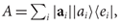where |ei〉 is an orthonormal basis, and the |ai〉 are normalized to 1. Assume that we have quantum access to the columns |ai〉 of A and to their norms |ai|. That is, we have a quantum computer or quantum random access memory18,19,20 that takes |i〉|0〉|0〉 → |i〉|ai〉||ai|〉. Quantum random access memory requires O(d) hardware resources to store all of the coefficients of the vectors and O(d) switches to make them accessible but allows access to the data in O(log d) operations. As in ref. 21, quantum access to vectors and norms allows us to construct the (unnormalized) state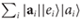: the density matrix for the second register is proportional to Σ. Using n = O(t2ε−1) copies of Σ/trΣ allows us to implement eitΣ/trΣ to accuracy ε in time O(n log d). Our method allows us to exponentiate any low-rank matrix Σ in time O(log d) as long as it is presented to us in Gram form Σ = AA, and we are given quantum access to the columns of A. In contrast, existing methods using the higher order Suzuki–Trotter expansion11,12,13,14 require O(d log d) operations to exponentiate non-sparse Hamiltonians. Density matrix exponentiation extends the efficient implementation of eiΣt to a large class of non-sparse but low-rank matrices Σ.

qPCA for quantum states can be extended to quantum processes by using the Choi–Jamiolkowski state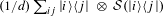for a completely positive map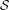(ref. 22). For a comprehensive review of the Choi–Jamiolkowski isomorphism in the context of quantum state and process tomography see ref. 2, including a detailed resources analysis of various entanglement-assisted protocols. For quantum channel tomography, for example, the Choi–Jamiolkowski state is obtained by sending half of a fully entangled quantum state down the channel. qPCA can then be used to construct the eigenvectors corresponding to the dominant eigenvalues of this state: the resulting spectral decomposition in turn encapsulates many of the most important properties of the channel22.

qPCA is a new state- and process-tomography primitive that reveals eigenvectors and eigenvalues of density matrices. To get a clearer picture of the advantages and disadvantages of qPCA, it is useful to compare it with quantum compressed sensing3,4,5,7,8, a powerful method for performing tomography on sparse and low-rank density matrices. The primary difference is that qPCA constructs eigenvectors and associates them with the corresponding eigenvalues in time O(R log d): the eigenvectors are then available in quantum form so that their properties can be tested by measurement, and correlated with the eigenvalues. Compressed sensing, in contrast3,4,5,7,8, is a state- and process-tomography method that reconstructs a classical description of the full density matrix in time O(Rd log d). Only single-qubit preparations and measurements are employed. qPCA could also be used to perform state tomography on the eigenvectors, revealing their components in time O(Rd log d). This classical description of the eigenvalues and eigenvectors can then be used to reproduce the full density matrix in time O(Rd log d), the same as in quantum compressed sensing but relying on the multi-qubit infinitesimal swap operation. In contrast, to construct the eigenvectors and eigenvalues using compressed sensing one must first reconstruct the density matrix and then diagonalize it, which takes time >O(d2logR + dR2) by randomized algorithms for low-rank matrices6. It can be shown by information-theoretic arguments that finding a low-rank approximation by sampling without prior knowledge is lower bounded by Ω(d) (ref. 23). qPCA can be compared to group-representation-based methods for estimating the spectrum and eigenvectors of a density matrix24,25,26. These methods reveal the spectrum24,25,26 in time O(poly(log d)) but take time O(d2) to reconstruct the eigenvectors26.

qPCA can also be useful in state discrimination and assignment27. For example, suppose that we can sample from two sets of m states, the first set {|ϕi〉} characterized by a density matrix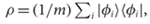and the second set {|ψi〉} characterized by a density matrix σ = (1/m)∑ i|ψi〉〈ψi|. Now we are given a new state |χ〉. Our job is to assign the state to one set or the other. Density matrix exponentiation and quantum phase estimation then allow us to decompose |χ〉 in terms of the eigenvectors and eigenvalues of the ρσ: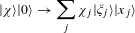where |ξj〉 are the eigenvectors of ρσ and xj are the corresponding eigenvalues. Measure the eigenvalue register, and assign |χ〉 to the first set if the eigenvalue is positive and to the second set if it is negative. If |χ〉 is selected from one of the two sets, this procedure is simply minimum error state discrimination1, but exponentially faster. As a bonus, the magnitude of the measured eigenvalue is a measure of the confidence of the set assignment measurement: larger magnitude eigenvalues correspond to higher confidence in the assignment, and magnitude 1 corresponds to certainty—in this case |ξ〉 is orthogonal to all of the members of one of the sets. If |χ〉 is some other vector, then the method provides a method for supervised learning and cluster assignment9,10,21,28: the two sets are training sets and the vector is assigned to the set of vectors to which it is more similar.

## Discussion

Density matrix exponentiation represents a powerful tool for analysing the properties of unknown density matrices. The ability to use n copies of ρ to apply the unitary operator eiρt allows us to exponentiate non-sparse d-dimensional matrices to accuracy ε = O(t2/n), and to perform qPCA to construct the eigenvectors and eigenvalues of a low-rank matrix ρ in time O(R log d). Like quantum matrix inversion14, qPCA maps a classical procedure that takes time polynomial in the dimension of a system to a quantum procedure that takes time polynomial in the logarithm of the dimension. This exponential compression means that qPCA can reveal only a fraction of the full information required to describe the system. That particular fraction of information can be very useful, however, as the ability of density matrix exponentiation to reconstruct its principal components shows.

We anticipate that qPCA can play a key role in a variety of quantum algorithms and measurement applications. As the example of quantum cluster assignment shows, qPCA could be useful for speeding up machine learning problems such as clustering and pattern recognition8,9,20,26. The ability to identify the largest eigenvalues of a matrix together with the corresponding eigenvectors is potentially useful for the representation and analysis of large amounts of high-dimensional data.

## References

1. Nielsen, M. A. & Chuang, I. L. Quantum Computation and Quantum Information (Cambridge Univ. Press, 2000).

2. Mohseni, M., Rezakhani, A. T. & Lidar, D. A. Quantum-process tomography: Resource analysis of different strategies. Phys. Rev. A 77, 032322 (2008).

3. Gross, D., Liu, Y-K., Flammia, S. T., Becker, S. & Eisert, J. Quantum state tomography via compressed sensing. Phys. Rev. Lett. 105, 150401 (2010).

4. Liu, Y-K. Universal low-rank matrix recovery from Pauli measurements. Adv. Neural Inf. Proc. Sys. (NIPS) 24, 1638–1646 (2011).

5. Flammia, S. T., Gross, D., Liu, Y-K. & Eisert, J. Quantum tomography via compressed sensing: Error bounds, sample complexity and efficient estimators. New J. Phys. 14, 095022 (2012).

6. Liberty, E., Woolfe, F., Martinsson, P-G., Rokhlin, V. & Tygert, M. Randomized algorithms for the low-rank approximation of matrices. Proc. Natl Acad. Sci. USA 104, 20167–20172 (2007).

7. Shabani, A. et al. Efficient measurement of quantum dynamics via compressive sensing. Phys. Rev. Lett. 106, 100401 (2011).

8. Shabani, A., Mohseni, M., Lloyd, S., Kosut, R. L. & Rabitz, H. Estimation of many-body quantum hamiltonians via compressive sensing. Phys. Rev. A 84, 012107 (2011).

9. Bishop, C. M. Pattern Recognition and Machine Learning (Springer, 2006).

10. Murphy, K. P. Machine Learning: A Probabilistic Perspective (MIT Press, 2012).

11. Lloyd, S. Universal quantum simulators. Science 273, 1073–1078 (1996).

12. Aharonov, D. & Ta-Shma, A. Proc. 35th Annu. ACM Symp. on Theory of Computing 20–29 (ACM, 2003).

13. Berry, D. W., Ahokas, G., Cleve, R. & Sanders, B. C. Efficient quantum algorithms for simulating sparse hamiltonians. Commun. Math. Phys. 270, 359–371 (2007).

14. Wiebe, N., Berry, D. W., Hoyer, P. & Sanders, B. C. Simulating quantum dynamics on a quantum computer. J. Phys. A 43, 065203 (2010).

15. Harrow, A. W., Hassidim, A. & Lloyd, S. Quantum algorithm for linear systems of equations. Phys. Rev. Lett. 103, 150502 (2009).

16. Araújo, M., Feix, A., Costa, F. & Brukner, Č. Quantum circuits cannot control unknown operations. Preprint at http://arxiv.org/abs/1309.7976 (2013).

17. Nakayama, S., Soeda, A. & Murao, M. Universal implementation of projective measurement of energy. Preprint at http://arxiv.org/abs/1310.3047 (2013).

18. Giovannetti, V., Lloyd, S. & Maccone, L. Quantum random access memory. Phys. Rev. Lett. 100, 160501 (2008).

19. Giovannetti, V., Lloyd, S. & Maccone, L. Architectures for a quantum random access memory. Phys. Rev. A 78, 052310 (2008).

20. De Martini, F. et al. Experimental quantum private queries with linear optics. Phys. Rev. A 80, 010302(R) (2009).

21. Lloyd, S., Mohseni, M. & Rebentrost, P. Quantum algorithms for supervised and unsupervised machine learning. Preprint at http://arxiv.org/abs/1307.0411 (2013).

22. Choi, M-D. Completely positive linear maps on complex matrices. Lin. Alg. Appl. 10, 285–290 (1975).

23. Bar-Yossef, Z. Proc. 35th Annu. ACM Symp. on Theory of Computing 335–344 (ACM, 2003).

24. Keyl, M. & Werner, R. F. Estimating the spectrum of a density operator. Phys. Rev. A 64, 052311 (2001).

25. Bacon, D., Chuang, I. L. & Harrow, A. W. Efficient quantum circuits for Schur and Clebsch–Gordan transforms. Phys. Rev. Lett. 97, 170502 (2006).

26. Keyl, M. Quantum state estimation and large deviations. Rev. Math. Phys. 18, 19–60 (2006).

27. Helstrom, C. W. Quantum Detection and Estimation Theory (Academic, 1976).

28. Rebentrost, P., Mohseni, M. & Lloyd, S. Quantum support vector machine for big data classification. Preprint at http://arxiv.org/abs/1307.0471 (2013).

## Acknowledgements

This work was supported by DARPA, Google-NASA Quantum Artificial Intelligence Laboratory, AFOSR under a MURI program, and J. Epstein. The authors thank A. Childs and R. Kothari for helpful discussions.

## Author information

Authors

### Contributions

S.L., M.M. and P.R. contributed to the theoretical research described in the paper and the writing of the manuscript.

### Corresponding author

Correspondence to Seth Lloyd.

## Ethics declarations

### Competing interests

The authors declare no competing financial interests.

## Rights and permissions

Reprints and Permissions

• ### Neural network-based prediction of the secret-key rate of quantum key distribution

• Min-Gang Zhou
• Zhi-Ping Liu
• Zeng-Bing Chen

Scientific Reports (2022)

• ### Realizing quantum convolutional neural networks on a superconducting quantum processor to recognize quantum phases

• Johannes Herrmann
• Sergi Masot Llima
• Christopher Eichler

Nature Communications (2022)

• ### Review of some existing QML frameworks and novel hybrid classical–quantum neural networks realising binary classification for the noisy datasets

• N. Schetakis
• D. Aghamalyan
• M. Boguslavsky

Scientific Reports (2022)

• ### Restrictions on realizable unitary operations imposed by symmetry and locality

• Iman Marvian

Nature Physics (2022)

• ### Quantum machine learning for support vector machine classification

• S. S. Kavitha
• Narasimha Kaulgud

Evolutionary Intelligence (2022)

## Search